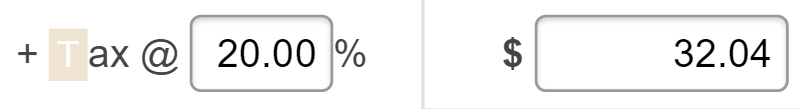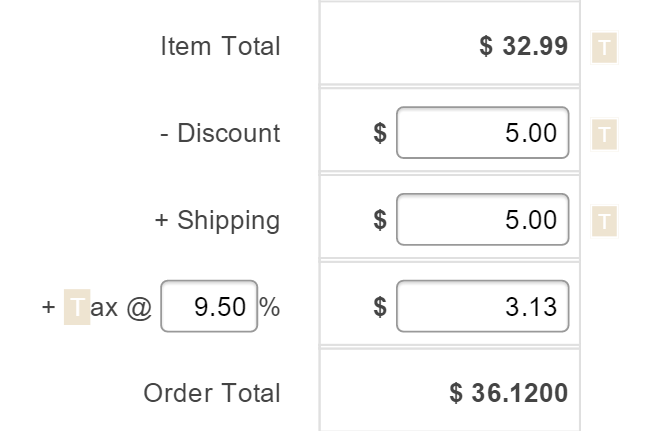Craftybase calculates your sales tax automatically for each order you create based on the Tax Rate you apply.

Your  Default Tax Rate set for your account is used as the default rate for each Order. You can also change your Tax Rate per Order, if need be. How do I set my Default Tax Rate?

### How do I set the tax rate for an Order?

To set the tax rate for an  Order:

1. Navigate to your Order (or create a new Order)
2. On the form, add the tax rate you wish to apply by completing the % text box next to the Tax total3. Save the order

### How do I add Tax for an Order?

Tax can be added as part of the calculated total for the Order by setting the Tax Rate for the Order or by entering the total tax amount in the text box (as shown below).Tax is calculated on the Item Total plus Shipping minus any Discount applied.### How do I add tax for an Order Line Item?

The total tax amount for the Order is automatically proportioned to each Order Line Item within it. This is so that tax amounts can be factored into your product level profitability calculations.

Proportioning tax is calculated based on the total cost of each item in the order.

As the Green Cushion’s total price represents 71% of the total item price of the order, the proportional tax is calculated as 71% of \$10 which is \$7.14.